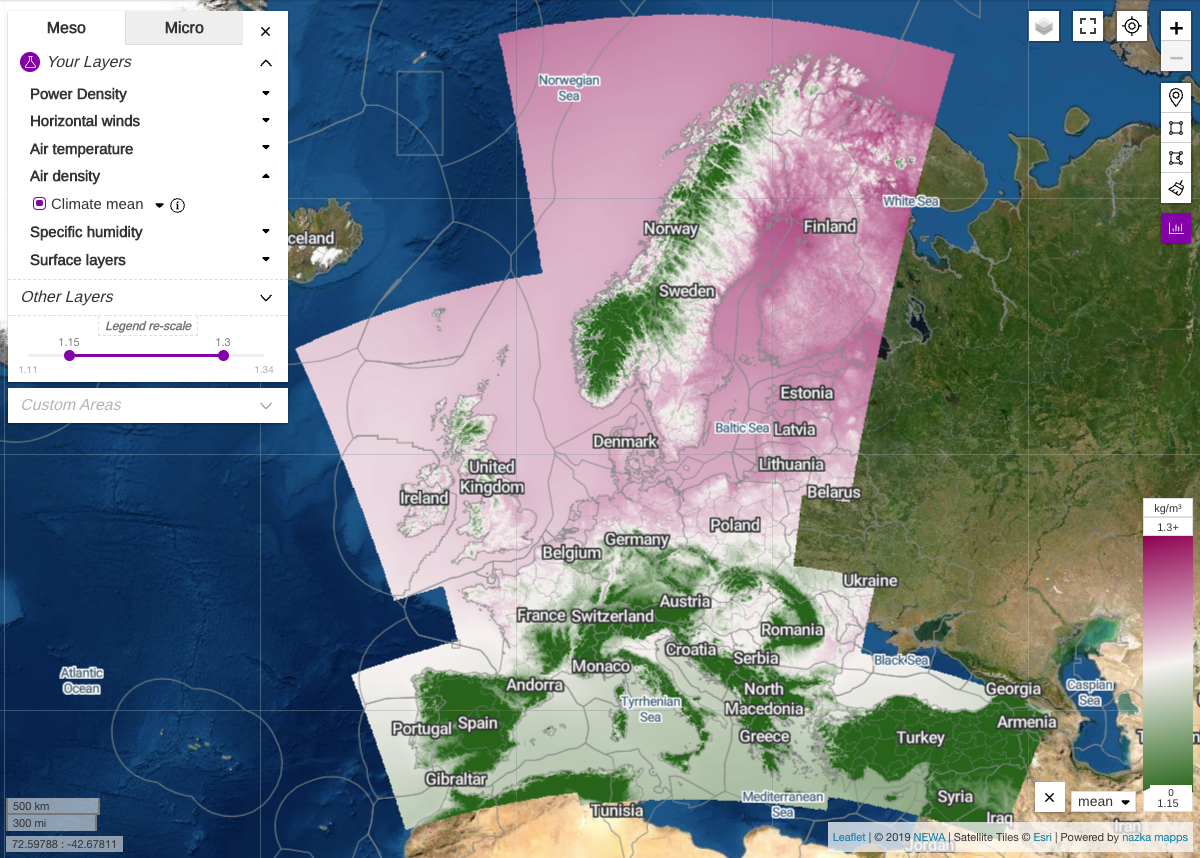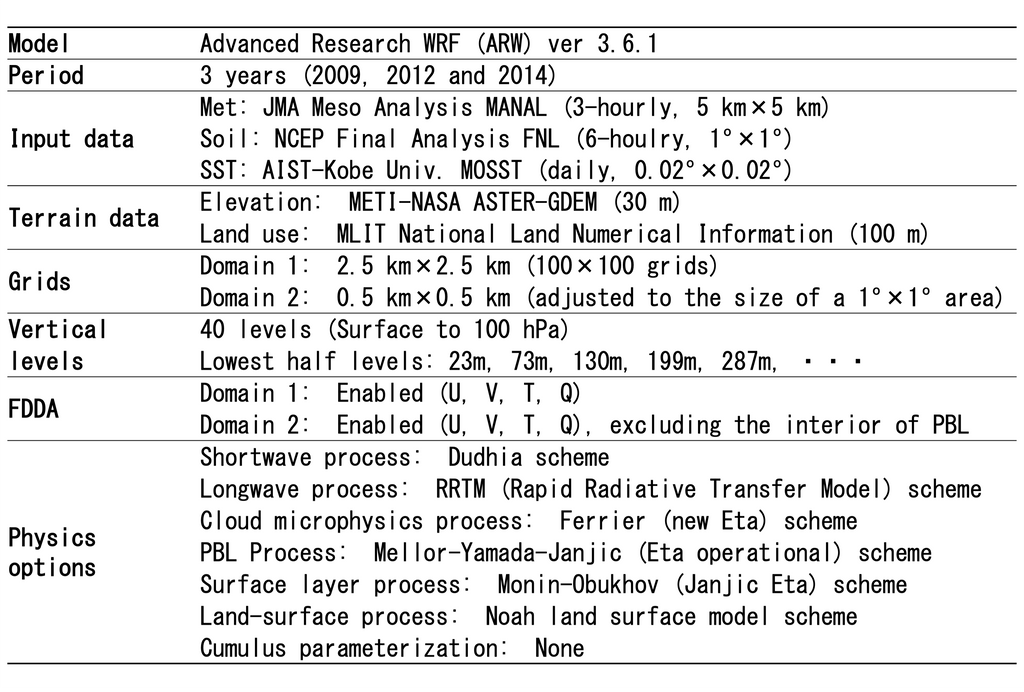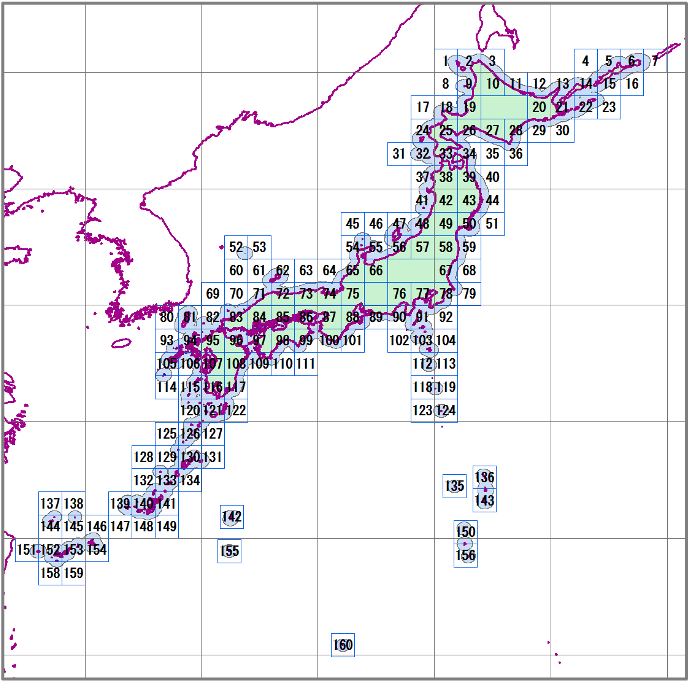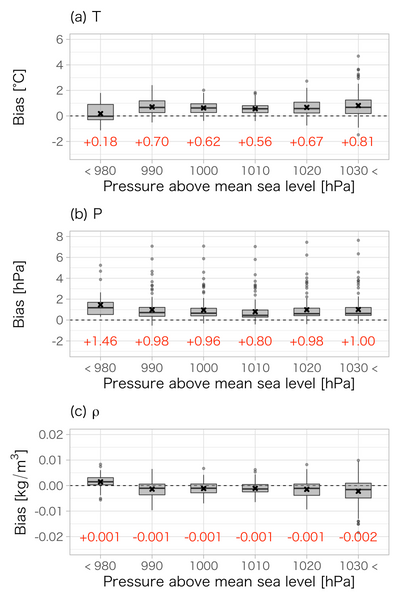# Accuracy verification of air density calculated by Meso -feeling model

This article is2021Year11Held in the month43This is a re -edited part of the "air density accuracy calculated by the meso -like model" announced at the symposium of the winding energy.

1. At the beginning

In the field of wind, air density is a weather element required for power generation evaluation and windmill design. For example, the "Wind Farm Certificate Authentication and Athletics Power Plant Edition" of the Japan Maritime Association1)Then, as a windmill design for windmill design, it is required to calculate the air density when power generation and storm waiting.

To get air density in a simple and wide area, Meso Mesae ModelWRFTHE WEATHER RESEARCH AND FORECASTING MODEL2) Simulation results with use) are useful (figure)1)is. However, it is important to verify the accuracy of these simulation results by referring to the observation value and understand and improve the error. So in this study,WRFThe accuracy of the air density calculated by using the observation value was verified, and the calculation error was considered.Figure 13)

2. Method

In this study, the offshore wind situation map NEOWINS4)Among the WRF simulation results used in the development of, a 1 -hour value of 500m lattice calculation for a remote distance of 30 km was used (Table 1 and Fig. 2). For verification of the WRF calculation results, the air density calculated using the temperature and air pressure of the Japan Meteorological Office (Fig. 3) at 142 domestic AMedas data of the Japan Meteorological Agency as a site observation value.

Table 1 WRF calculation condition4)Figure 2 WRF calculation area4)Figure 3 Position of 142 points of meteorological offices

3. Results and discussion

Fig. 4 shows a beard diagram of BIAS (average error) and R2 (determined coefficient) of the WRF calculation value of the WRF calculation value at the metal government office. The absolute value of BIAS is small, the average value of the determined coefficient is 0.97 to 0.99, and most of each sample varies. From these results, we can see that WRF calculates high temperature, pressure and air density at high precision.Figure 4 Figure 4 (A) temperature of WRF calculation values ​​in the meteorological office, (b), (c) air density (1) BIAS and (2) R2 beard diagram
* The values ​​at the bottom of the figure are the average of each statistical amount.

FIG. 5 shows the results of the Bias in FIG. When the sea level comparing spuream is larger than the 980 [HPA], BIAS matches the positive and negative tendency of all samples (Fig. 4). On the other hand, when the sea level comparison spuream was 980 [HPA] or less, the BIAS had a smaller temperature, the positive value of the air pressure, and the air density was slightly positive compared to other samples. 。 The result is a tendency for WRF to overestimate the air density by overestimating the air pressure when the air pressure is underway (when low pressure or typhoon passes). I suggest that.

In order to improve the accuracy of WRF air density, it is necessary to improve the error between temperature and air pressure. RTI plans to continue research, including these weather elements, with the aim of improving the accuracy of WRF simulation.Fig. 5 (A) temperature, (b), (c) air density BIAS Bias map of the WRF calculation value of sea level and air pressure in the meteorological office

* Each statistical amount is calculated by categorizing the sea level rectomic sparity observed at the Japan Meteorological Office for each bottle of 10 [HPA], and the statistics of less than 30 samples are excluded in advance. The value at the bottom of the figure is the average of each statistical amount.

4. summary

In this study, the Meteorological Agency's Amedas observation value is referred to, and the Meso Meteorological modelWRFVerified the calculation accuracy of air density calculated by. The main conclusions are summarized below.

• As a result of verification using the Amedas observation valueWRFHowever, it became clear that the air density could be reproduced with high accuracy.
• For the sea level correction air pressureWRFWhen comparing the calculation values, when low pressure or typhoon passes,WRFIt was suggested that overrated the air pressure to slightly overestimate air density.

References

1) Japan Maritime Association, Wind Farm Certificate Land Wind Power Station Edition, 2021, P.41, URL:https://www.classnk.or.jp/hp/pdf/authentication/renewableenergy/ja/windfarm/NKRE-GL-WFC01_July2021_Jpn_Corrected_October2021.pdf(access:2022February 2nd year).

2) SKAMAROCK, W., KLEMP, J., J., J., GILL, D., Barker, D., DUDA, DUDA, M., HUANG, WANG, WANG, POWERS, J. A Description of Thee Advanced Research WRF Version 3. NCAR Tech. Note Ncar/TN-475+ Str. 2008.

3) The Newa Project, URL:https://www.neweuropeanwindatlas.eu/(Access: January 11, 2022).

4) NEDO, offshore style mapNEOWINS, URL: http://app10.infoc.nedo.go.jp/Nedo_Webgis/top.html(access:2022February 2nd year).